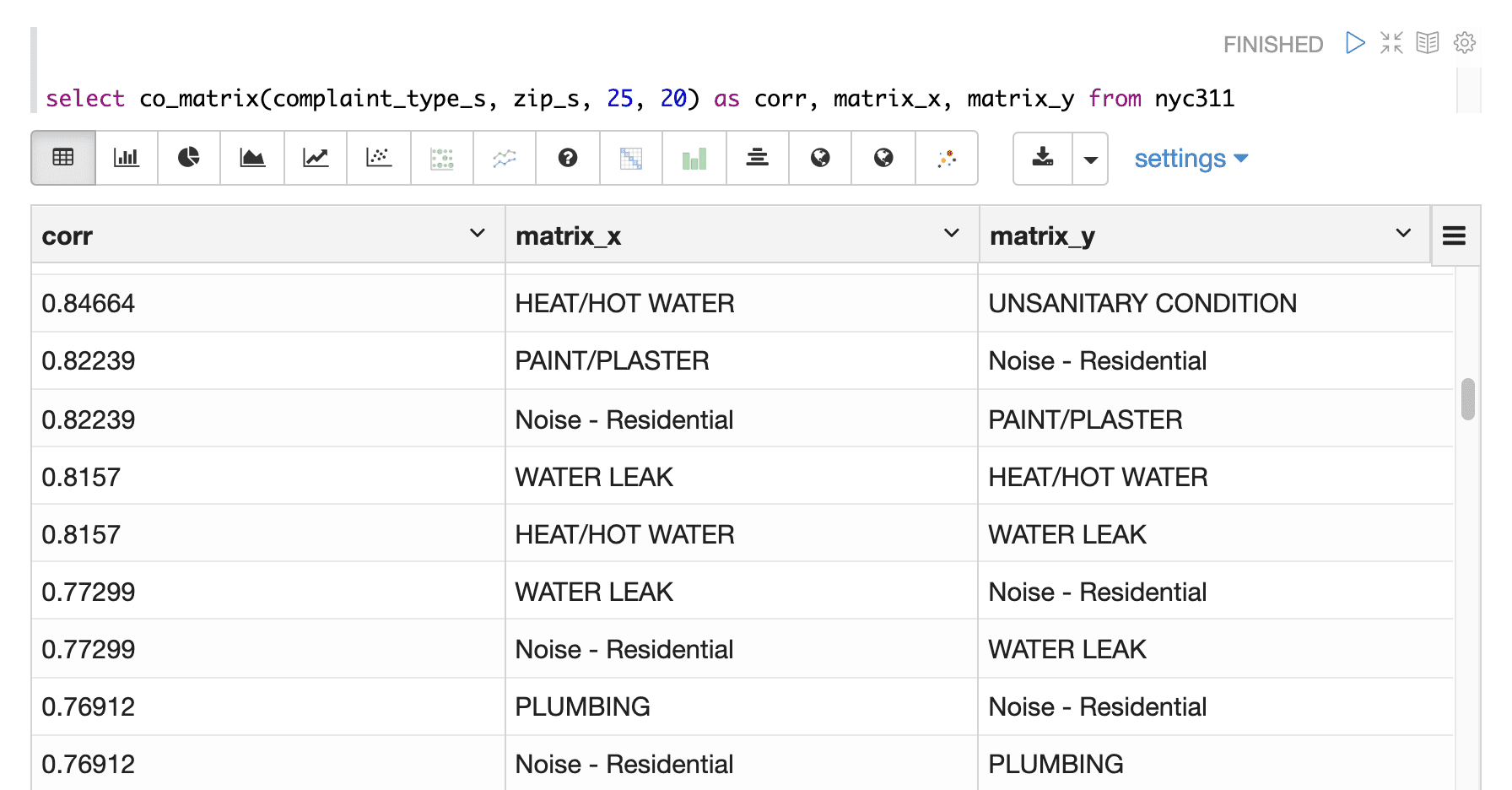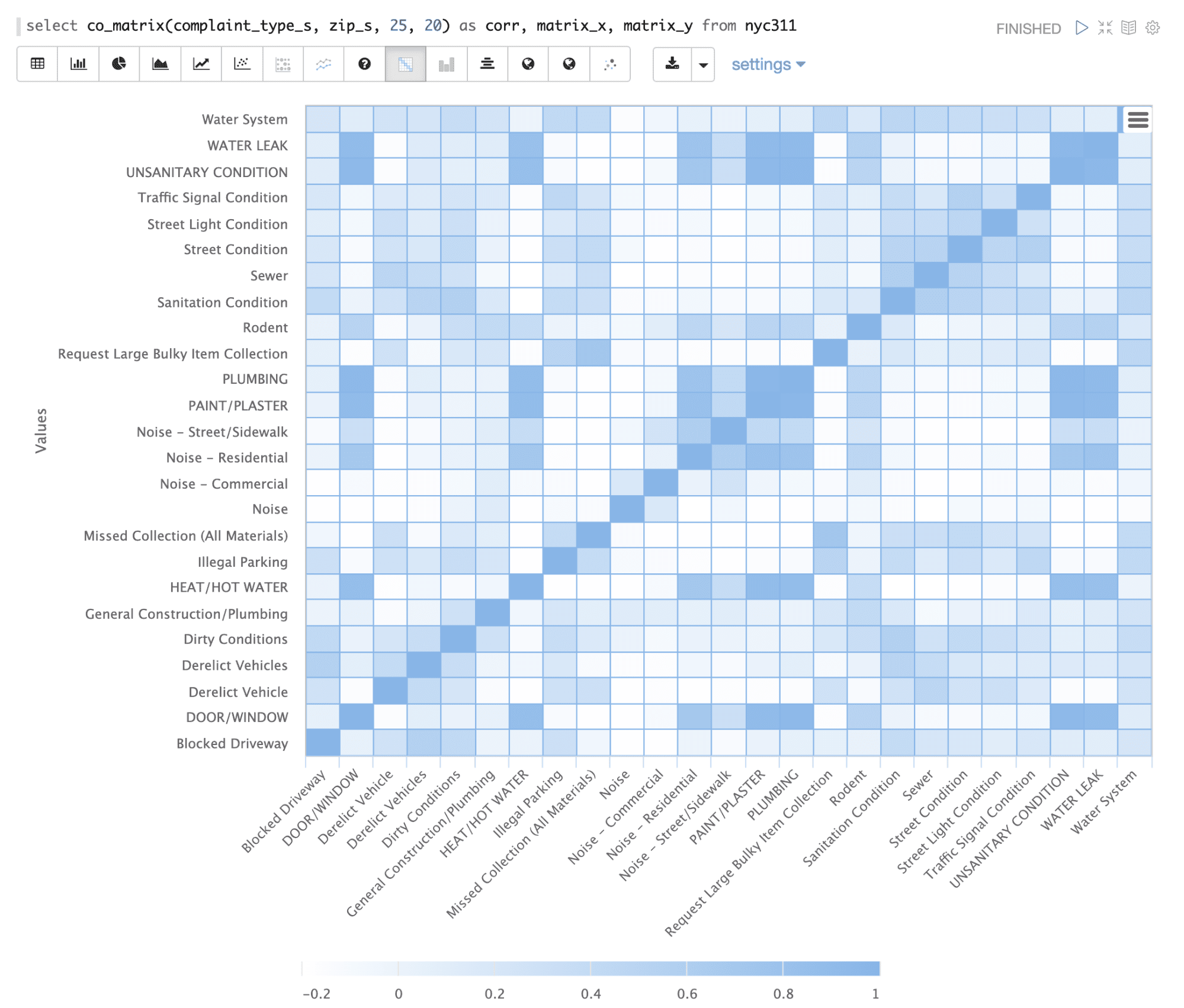# Co-occurrence Matrices (co_matrix)

The `co_matrix` function returns a matrix that shows the correlation of values within a categorical field based on their co-occurrence with another categorical field. For example in a medical database this could be used to correlate diseases by co-occurring symptoms. In the example below the `co_matrix` function is used to correlate complaint types across zip codes in the NYC 311 complaint database. This can be used to better understand how complaint types tend to go together. The `co_matrix` function has 4 parameters:

1. The categorical String field that will be correlated.

2. The categorical String field that will be used for co-occurrence.

3. Number of categorical variables to correlate.

4. Number of categorical variables to calculate co-occurrence from.

## Sample syntax:

In the example below the top 25 values in the `complaint_type_s` field are correlated across the top 20 values in the `zip_s` field in the NYC 311 complaint database.

``````select co_matrix(complaint_type_s, zip_s, 25, 20) as corr,
matrix_x,
matrix_y
from nyc311``````

## Result set

The result set for the `co_matrix` function is a correlation matrix for the first categorical field parameter. The `co_matrix` function returns the correlation for each row. The `matrix_x` and `matrix_y` fields contain the combinations of the top N categorical values.

In the example below Apache Zeppelin is used to display the the correlation matrix result for the top 25 occurring values in the `complaint_type_s` field:## Visualization

The `co_matrix` function can be visualized in a heatmap by plotting `matrix_x` on the x-axis, `matrix_y` on the y-axis and the correlation value in the cells. The example below shows the `co_matrix` function visualized in an Apache Zeppelin heatmap: